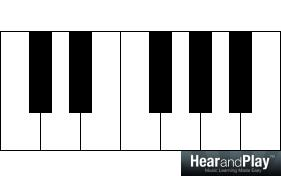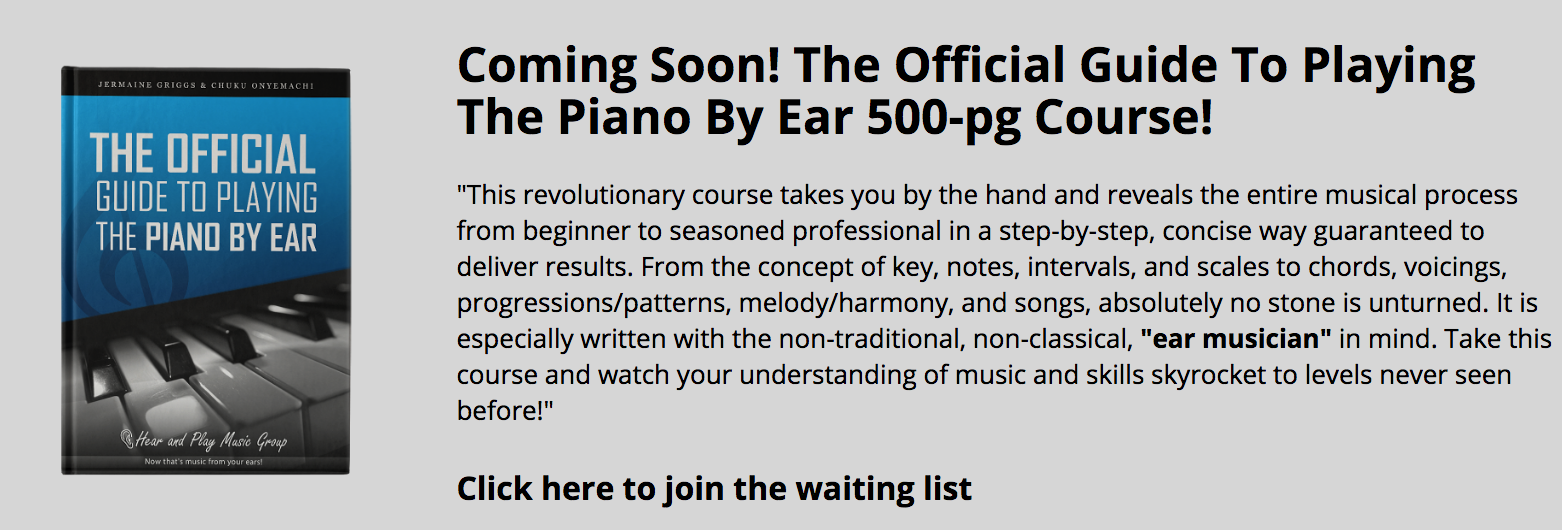• # The Relationship Between Diatonic Dissonant Intervals

inDissonance is the inharmonious outcome of the relationship between two notes heard melodically (one after the other) or harmonically (together).

When any idea (note, scale, interval, chord, chord progression, etc.) is said to be diatonic, it means the idea is a product of the relationship between the 7 natural tones of any key (without accidentals).

When the relationship between two notes of the scale is inharmonious, such dissonance is known as diatonic dissonance. Some of these intervals may sound harsh like their chromatic counterparts but they are not FORBIDDEN in traditional practice because they are inevitable (they occur between natural tones).

Below are diatonic dissonant intervals:

Major Second

Minor second

Major Seventh

Minor seventh

As you might notice, there are two qualities and two quantities of diatonic dissonant intervals. Let’s look at both distinctions.

Quantity          –           Second | Seventh

Quality            –           Major    |   Minor

There are two widths or quantities of intervals that are associated with diatonic dissonance (seconds and sevenths) and two qualities (major and minor). Therefore, we’ll be using seconds/sevenths and major/minor a lot in this article.

The following diatonic intervals (built on C major) are  dissonant intervals:

Major Second (between the 2nd and 3rd scale steps)Minor second (between the 3rd and 4th scale steps)Major Seventh (between the 1st and 7th scale steps)Minor seventh (between the 2nd and 1st scale steps)These are all examples of diatonic dissonant intervals.

## THE RELATIONSHIP BETWEEN DIATONIC DISSONANT INTERVALS.

Diatonic dissonant intervals are related by the simple process of inversion.  After the process of inversion, intervals change. There’s change in both quality and quantity. Let’s explore these changes and the relationship between diatonic dissonant intervals.

### Relationship #1 – Quantity

Intervals can be described using numbers that are equivalent to the number of letter names they encompass. Let’s look at the two quantities of diatonic dissonant intervals:

Intervals described as seconds span two letter names. For example, C-D:D-E:E-F (etc.):Intervals that encompass seven letter names are called sevenths.

C-B (C D E F G A B):D-C (D E F G A B C):E-D (E D F G A B C D):etc.

The simple process of inversion and its effect on the quantity of an interval is the relationship that binds these two quantities together. Let me elucidate further by analyzing the inversion of these widths.

#### Inversion of Seconds

Intervals can be inverted using the octave transposition technique. Therefore, seconds like C-D can be inverted by transposing either the melody note an octave lower or the bass note an octave higher. For example:C-D: C is the bass note and D is the melody note.

Approach #1Octave transposition of C to a higher octave will yield D-C.Approach #2 Octave transposition of D to a lower octave will also yield D-C.Now, that we’ve inverted C-D into D-C, what is the size of D-C?D-C encompasses D, E, F, G, A, B and C, which is seven letter names.

Therefore, inversion of a second (C-D) will yield a seventh (D-C). It works on any second in any scale, every time.

#### Inversion of Sevenths

Sevenths like C-B can be inverted in the same way by transposing either the melody note an octave lower or the bass note an octave higher.C-B: C is the bass note and B is the melody note.

Approach #1Octave transposition of C to a higher octave will yield B-C.Approach #2 Octave transposition of B to a lower octave will also yield B-C.Now, that we’ve inverted C-B into B-C, what is the size of B-C?B-C encompasses B and C, which is two letter names.

Therefore, inversion of a seventh (C-B) will yield a second (B-C).

### Relationship #2 – Quality

Intervals can be described using adjectives that correspond to their harmonic property. Let’s look at the two qualities of diatonic dissonant intervals:

Major intervals are intervals that are built off the scale tones of the bass note.

Considering that C is our bass note, the second and seventh scale tones of C Major scale will yield major intervals.

C-D (1st and second scale tones):C-B (1st and seventh scale tones):Using the key of the bass note, minor intervals are intervals that are built off non-scale tones. Minor intervals are usually lower than major intervals by a semitone progression (or half step).

C-D♭:C-B♭:The simple process of inversion also has its effect on the quality of an interval, just as we witnessed in the previous section concerning quantities (seconds and sevenths).

Let me show you step-by-step how this works.The quality of the interval C-B, is determined by the major scale of C (which is the bass note).Therefore, considering that B is, indeed, a scale tone of the C major scale, it is described using the major quality. Therefore C-B is a major interval.

Alternatively, if we have C-B:…using the same scale of C:We have a B note that is a non-scale tone and is also lower than the seventh scale step by a semitone (or half step).

Therefore, C-B is a minor interval.The relationship between these two qualities (major and minor) is inversion. The simple process of inversion binds these two qualities together. Another way to say it is: “They are inversions of each other.”

#### Inversion of Major Intervals

Using the octave transposition technique, an interval of a major second (C-D) can be inverted by transposing either the melody note an octave lower or the bass note an octave higher.C-D: C is the bass note and D is the melody note.

Approach #1 – Octave transposition of C to a higher octave will yield D-C.Approach #2 – Octave transposition of D to a lower octave will also yield D-C.Now, that we’ve inverted C-D into D-C, what is the quality of D-C?

The quality of D-C will be determined using the major scale of D (its new bass note):C is not a scale tone of the D major scale above (it’s a C). It is lower than C by a semitone progression (or half step).

Therefore, D-C is a minor interval.

*Inversion of a major interval (C-D) will yield a minor interval (D-C) every time.

#### Inversion of Minor Intervals

The minor second (C-D) can be inverted by transposing either the melody note an octave lower or the bass note an octave higher.C-D: C is the bass note and D is the melody note.

Approach #1 – Octave transposition of C to a higher octave will yield D-C.Approach #2 – Octave transposition of D to a lower octave will also yield D-C.Now, that we’ve inverted C-D into D-C, what is the quality of D-C?

The quality of D-C will be determined by the major scale of D (its new bass note).C is the seventh scale tone in the D major scale above

Therefore, D-C is a major interval.

*Inversion of a minor interval (C-D) will yield a major interval (D-C) every time.

Before we go any further, here’s what we’ve derived so far:

1st Relationship – All seconds and sevenths are related by inversion of quantity.

This means that all intervals of seconds are potential sevenths. This potential is harnessed by inversion. Inversion transforms all seconds to sevenths. The same thing applies to sevenths – they are potential seconds.

2nd Relationship – All major and minor intervals are related by inversion of quality.

Inversion can change all major intervals to minor intervals and vice versa. Therefore, all major intervals are potential minor intervals and all minor intervals are potential major intervals.

Let’s look at the outcome of inverting dissonant intervals in terms of quality and quantity.

Outcome #1 – Major second and minor seventh intervals are related by inversion.

Inversion of a Major 2nd

Inversion of Quality: Major becomes minor.

Inversion of Quantity: 2nd becomes 7th.

**Inversion of a major 2nd yields a minor 7th.

If we reverse the process:

Inversion of a Minor 7th

Inversion of Quality: Minor becomes major.

Inversion of Quantity: 7th becomes 2nd.

**Inversion of a minor 7th yields a major 2nd.

Outcome #2 – Minor second and major seventh intervals are related by inversion.

Inversion of a Minor 2nd

Inversion of Quality: Minor becomes major.

Inversion of Quantity: 2nd becomes 7th.

**Inversion of a minor 2nd yields a major 7th.

If we reverse the process:

Inversion of a Major 7th

Inversion of Quality: Major becomes minor.

Inversion of Quantity: 7th becomes 2nd.

**Inversion of a major 7th yields a minor 2nd.

We’ll definitely learn more about the practical applications of these intervals in our future lessons on chord formations.

Until then!

The following two tabs change content below.#### Chuku Onyemachi

Head of Education at HearandPlay Music Group
Onyemachi "Onye" Chuku (aka - "Dr. Pokey") is a Nigerian musicologist, pianist, and author. Inspired by his role model (Jermaine Griggs) who has become his mentor, what he started off as teaching musicians in his Aba-Nigeria neighborhood in April 2005 eventually morphed into an international career that has helped hundreds of thousands of musicians all around the world. Onye lives in Dubai and is currently the Head of Education at HearandPlay Music Group and the music consultant of the Gospel Music Training Center, all in California, USA.#### Latest posts by Chuku Onyemachi (see all)